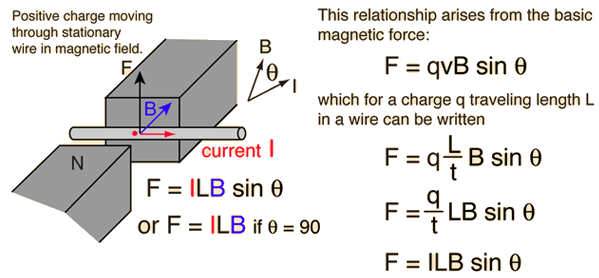Home » 2012 » June » 18

# Daily Archives: June 18, 2012

## Force on a current carrying conductor in a magnetic field

Why the force acting on current carrying conductor when placed in a magnetic field increases with the increase in the current.?

I mean what happens inside it at that time? Please explain it in detail, it’s really confusing me, Please answer it as sson as possible.

Let’s consider the terms involved here first.• Electric Current is the rate of flow of charges. An increase in current through a conductor means more number of electrons are crossing any cross section of the wire per second.
• A moving charge experience a force in magnetic field because a moving charge creates a magnetic field around it. The two interacting magnetic fields causes the force.

So, let’s summarize, when there is a current flowing through a conductor placed in a magnetic field, it experiences a force due to the mutual interaction between the magnetic fields – the already existing field and the field produced by the motion of current carriers (free electrons) inside the conductor.

When there is an increase in current, the force of interaction also increase as the numbers of free electrons flowing through any cross section also increases.

## A thought provoking (HOTS) problem from rotation

Consider a disk rotating in a horizontal plane with a constant angular speed “omega” about its center O.

The disk has a shaded region on one side of the diameter and an unshaded region on the other  side.

When disk is in horizontal plane x-y with left half shaded and right half unshaded, two pebbles P and Q are simultaneously projected at an angle towards R.

The velocity of projection is in the y-z plane and is same for both pebbles with respect to the disk.

Assume that

(i) they land back on the disk before the disk has completed one-eighth rotation,

(ii) their range is less than half the disk  radius,and

(iii)”omega” remains constant throughout.

Then where will the two pebbles land?

(All are invited to answer this)

## A doubt from Dimensional Analysis

st=u+0.5a(2t-1), both dimensionally or any other

The equation refers to the displacement in the tth second and hence it should have the dimensions of speed; since it is the displacement in one second.

Under this consideration, the equation is dimensionally and otherwise correct.

## Thoughts on Higgs Boson

When it is not contested scientifically that change is a continuous phenomenon,how is that Higgs boson, if found,can be considered as the ultimate building block of matter.

Sure, it may prove a mathematically arrived conclusion… yet the contradiction remains.
An ever changing, at the same time, ever stable (because it is the ultimate one) is the contradiction.
One may find changing or divisible particle only…

(Posted by K.C. Muralidhar)